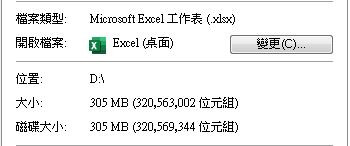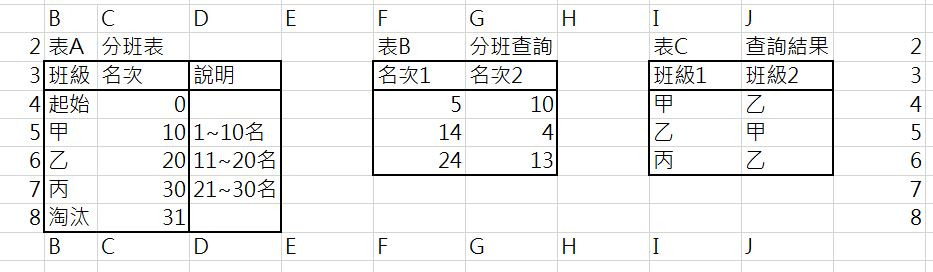#0

## Excel 有辦法將公式轉換成 VBA，以減少檔案大小，進而增加開啟速度嗎？step1. 將表 B儲存格 A1的值 =0.487971，在表 A的 D欄的值比對，

step2. 依據 step1結果，找到表 A的 D15對應在 A欄的位置 A15，再+1=A16。

step3. 將表 A儲存格 A16的值，填入表 C的 A2儲存格。

froce iT邦大師 1 級 ‧ 2019-07-07 11:07:35 檢舉

froce iT邦大師 1 級 ‧ 2019-07-07 12:54:54 檢舉

froce iT邦大師 1 級 ‧ 2019-07-07 14:33:28 檢舉

froce iT邦大師 1 級 ‧ 2019-07-07 17:04:54 檢舉

Access是比較易用的有GUI介面的資料庫，但如果貴校有開什麼供學生去學的python資料分析課程，我是更建議去聽一聽。

python好像是當今顯學，身邊也是有朋友去學網路課程，意圖分析股市投資策略。就找時間來學看看了...
goodnight iT邦研究生 3 級 ‧ 2019-07-09 18:18:28 檢舉
excel 2010 以上的版本, 效率都很好了, 以 2016 版最好

goodnight iT邦研究生 3 級 ‧ 2019-07-09 18:21:41 檢舉

goodnight iT邦研究生 3 級 ‧ 2019-07-10 14:46:47 檢舉

goodnight iT邦研究生 3 級 ‧ 2019-07-10 16:04:21 檢舉

step1. 將表 B儲存格 A1的值 =0.487971，在表 A的 D欄的值比對，找到（表B, A1）=0.487971 >（表A, D15）=0.446153846。

=IFERROR(INDEX(A!\$A\$2:\$A\$100,MATCH(B!A1,A!\$D\$2:\$D\$100)+1,1),A!\$A\$3)

1. MATCH(B!A1,A!\$D\$2:\$D\$100)+1,1)

2. INDEX(A!\$A\$2:\$A\$100,MATCH(B!A1,A!\$D\$2:\$D\$100)+1,1)

3. IFERROR(INDEX(A!\$A\$2:\$A\$100,MATCH(B!A1,A!\$D\$2:\$D\$100)+1,1),A!\$A\$3)

1

### 解決方法``````Sub 跨資料表查詢()

'宣告
'資料表設定：表A,表B,表C
Dim shA, shB, shC, fml As String
shA = "A"
shB = "A"
shC = "A"

'公式設定
fml = "=INDEX(\$B\$5:\$B\$9,MATCH(F5,\$C\$5:\$C\$9)+1,1)"

'輸出範圍
Set result = Sheets(shC).Range("I5: J7")

'處理過程
endRow = result.Rows.Count
result.Rows(1).Formula = fml

For reRow = 2 To endRow
result.Rows(1).Copy

With result.Rows(reRow)
.PasteSpecial
.Copy
.PasteSpecial Paste:=xlPasteValues
End With
Next reRow

With result.Rows(1)
.Copy
.PasteSpecial Paste:=xlPasteValues
End With

End Sub
``````

``````Sub FindValue()

Dim SearchRange As Range
Dim ARow As Long
Dim BRow As Long
Dim BCol As Long
Dim CRow As Long
Dim CCol As Long
Dim BEndRow As Long
Dim BEndCol As Long

Set SearchRange = Sheets("A").Range("D2:D30")      ' 在表 A要查找的範圍

BCol = 1
CCol = 1

EndBCol = Range("A1").End(xlToRight).Column      ' 找表 B最後一欄的資料

Do Until BCol > EndBCol

BRow = 1
CRow = 2

EndBrow = Sheets("B").Range("A1").End(xlDown).Row      ' 找表 B最後一列的資料

Do Until BRow > EndBrow

For Each cell In SearchRange
If cell.Value > Sheets("B").Cells(BRow, BCol).Value Then
ARow = cell.Row     ' 找(表 A, D2:D30) >表 B儲存格的值的位置
Exit For
End If

Next

Sheets("C").Cells(CRow, CCol) = Sheets("A").Range("A" & ARow).Value     '把表 A, A欄的值填入表 C

BRow = BRow + 1
CRow = CRow + 1

Loop

BCol = BCol + 1
CCol = CCol + 1

Loop

End Sub

``````

0

"來杯拿鐵"的比較符合你要的答案, 我是來串門子練功夫

``````Sub MAIN()

Dim rng As Object '物件
Dim dataRow As Integer '表B 的資料列數
Dim dataCol As Integer '表B 的資料欄數
Dim xRow As Integer '表B列, 用來循序讀取列資料
Dim xCol As Integer '表B欄, 用來循序移動欄座標讀取資料
Dim fData As Double '要比對的表B值
Dim fRow As Integer '比對表A值, 符合條件的列數
Dim dataEnd As Integer '表A 的資料列數
Dim cRow As Integer '要寫入表C 的列的位置

Application.ScreenUpdating = False ' 關閉螢幕的顯示

'--------------------------------------------------
'填入表C 的標題列, 有點多餘, 看你要不要用
Worksheets("表C").Range("A1").Value = "1A"
Worksheets("表C").Range("B1").Value = "1B"
Worksheets("表C").Range("C1").Value = "1C"
Worksheets("表C").Range("D1").Value = "1D"
Worksheets("表C").Range("E1").Value = "1E"
'--------------------------------------------------

' 切換到表A, 取得最後的資料列位置
Worksheets("表A").Activate
dataEnd = Cells(ActiveSheet.Rows.Count, "D").End(xlUp).Row

' 切換到表B 最後的資料列及欄位數
Worksheets("表B").Activate
dataRow = Cells(ActiveSheet.Rows.Count, "A").End(xlUp).Row ' 取得資料最後列
dataCol = Cells(1, ActiveSheet.Columns.Count).End(xlToLeft).Column ' 取得資料最右欄

For xCol = 1 To dataCol '表B 欄
cRow = 1 '表C 的欄題列值, 0表示沒有標題列
For xRow = 1 To dataRow ' 表B 列
' 取得表B RANGE(A1~A最後列)的值, CELLS(列, 欄), 例 CELLS(1, "A") = A1 或 CELLS(1,1) = A1
fData = Worksheets("表B").Cells(xRow, xCol).Value

' 開始比對資料
Worksheets("表A").Activate
For Each rng In Range("D2:D" & dataEnd)

If rng.Text > fData Then
fRow = rng.Row ' 符合比對條件的資料列數值
cRow = cRow + 1 ' 寫入表C的列數值
' 把指定的表A值填入表C
Worksheets("表C").Cells(cRow, xCol).Value = Worksheets("表A").Cells(fRow, "A").Value
Exit For '結束此次比對
End If
Next

Worksheets("表B").Activate
Next xRow
Next xCol

Application.ScreenUpdating = True

End Sub
``````
goodnight iT邦研究生 3 級 ‧ 2019-07-10 16:39:59 檢舉

``````Worksheets("表C").Select
Range("A1").Select
Application.ScreenUpdating = True
``````

goodnight iT邦研究生 3 級 ‧ 2019-07-10 20:56:39 檢舉